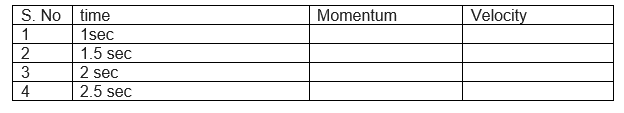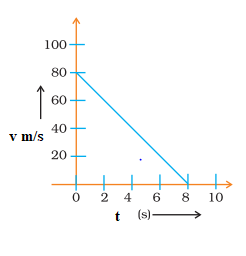# Force & Laws Of Motion Numericals Chapter 9 Class 9 Physics

Given below are the force and laws of motion class 9 numericals
(a) Objective Type Questions
(b) Table Type Questions
(c) Very Short questions
(c) Short questions
Hope you like them and do not forget to like , social share and comment at the end of the page.

## Objective Type Questions

Question 1
If you apply a net force of 3 N on .1 kg-box, what is the acceleration of the box
(a) 2 m/s2
(b) 30 m/s2
(c) 10 m/s2
(d) None of these.

Question 2
If a net force of 7 N was constantly applied on 400 g object at rest, how long will it take to raise its velocity to 80 m/s?
a. 0 s
b. 2.23 s
c. 3.47 s
d. 4.57 s

Question 3
An object of mass 10 g is sliding with a constant velocity of 2 m/ s on a frictionless horizontal table. The force required to keep the object moving with the same velocity is
(a) 0 N
(b) 5 N
(c) 10 N
(d)20 N

Question 4
An object of mass 2 kg is sliding with a constant velocity of 8 m/s on a frictionless horizontal table. The force required to keep the object moving with the same velocity is
(a) 16 N
(b) 8 N
(c) 2 N
(d) 0 N

## Table type Question

Question 5.
A particle of 10 kg is moving in a constant acceleration 2m/s2 starting from rest. What is its momentum and velocity per the table given belowQuestion 6
How much net force is required to accelerate a 1000 kg car at 4.00 m/s2?

Question 7
A body of mass 1 kg undergoes a change of velocity of 4m/s in 4s what is the force acting on it?

Question 8
A sedan car of mass 200kg is moving with a certain velocity . It is brought to rest by the application of brakes, within a distance of 20m when the average resistance being offered to it is 500N.What was the velocity of the motor car?

Question 9
A driver accelerates his car first at the rate of 4 m/s2 and then at the rate of 8 m/s2 .Calculate the ration of the forces exerted by the engines?

Question 10
A cricket ball of mass 0.20 kg is moving with a velocity of 1.2m/s . Find the impulse on the ball and average force applied by the player if he is able to stop the ball in 0.10s?

Question 11
A hockey ball of mass .2 Kg travelling at 10 ms-1 is struck by a hockey stick so as to return it along its original path with a velocity at 2 m/s . Calculate the change of momentum occurred in the motion of the hockey ball by the force applied by the hockey stick.

Question 12
Two objects of masses of 100 gm and 200 gm are moving in along the same line and direction with velocities of 2 ms-1 and 1 ms-1 respectively. They collide and after collision, the first object moves at a velocity of 1.67 ms-1. Determine the velocity of the second object?

Question 13
Anand leaves his house at 8.30 a.m. for his school. The school is 2 km away and classes start at 9.00 a.m. If he walks at a speed of 3 km/h for the first kilometre, at what speed should he walk the second kilometre to reach just in time?

Question 14
An object of mass 1kg acquires a speed of 10 m/s when pushed forward. What is the impulse given to the object?

Question 15
A bullet of mass 10 gm is fired with an initial velocity of 20 m/s from a rifle of mass 4 kg. Calculate the initial recoil velocity of the rifle?

Question 16
Which would require a greater force �� accelerating a 2 kg mass at 5 m/s2 or a 6 kg mass at 2 m/s2?

Question 17.
A force of 5 N produces an acceleration of 8 m/s2 on a mass $m_1$ and an acceleration of 24 m/s2 on a mass $m_2$. What acceleration would the same force provide if both the masses are tied together?

Question 18.
A hammer of mass 500 g, moving at 50 m/s, strikes a nail. The nail stops the hammer in a very short time of 0.01 s. what is the force of the nail on the hammer?

Question 19
An 8000 kg engine pulls a train of 5 wagons, each of 2000 kg along a horizontal track. If the engine exerts a force of 40000 N and the track offers a friction force of 5000 N, then calculate:
(a) The net accelerating force;
(b) The acceleration of the train; and
(c) The force of wagon 1 on wagon 2.

Question 20.
A bullet of 10 g strikes a sand bag at a speed of 103 m/s and gets embedded after travelling 5 cm. Calculate
(i) the resistive force exerted by the sand on the bullet.
(ii) the time taken by the bullet to come to rest.

Question 21.
Two objects A and B, having mass 100 kg and 75 kg, moving with velocity 40 km/hr and 6 km/hr respectively. Answer the following:
a.Which will have greater inertia?
b.Which will have greater momentum?
c.Which will stop first if equal negative acceleration is applied on both?
d.Which will travel greater distance?
e.Which will impart greater impulse if collides with a wall?

Question 22
A car start from rest and acquire a velocity of 54 km/h in 2 sec. Find
(i) the acceleration
(ii) distance travelled by car assume motion of car is uniform
(iii) If the mass of the car is 1000 Kg,what is the force acting on it?

Question 23
Velocity versus time graph of a ball of mass 100 g rolling on a concrete floor is shown below. Calculate the acceleration and the frictional force of the floor on the ball?Question 24.
A bullet of mass 10 g moving with a velocity of 400 m/s gets embedded in a freely suspended wooden block of mass 900 g. what is the velocity acquired by the block?

Question 25.
A man weighing 60 kg runs along the rails with a velocity of 18 km/h and jumps into a car of mass 1 quintal (100 kg) standing on the rails. Calculate the velocity with which car will start travelling along the rails.

## Summary

This numericals on force and laws of motion for class 9 is prepared keeping in mind the latest syllabus of CBSE . This has been designed in a way to improve the academic performance of the students. If you find mistakes , please do provide the feedback on the mail.